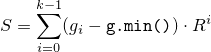# 26 Numerics library [numerics]

## 26.5 Random number generation [rand]

### 26.5.7 Utilities [rand.util]

#### 26.5.7.2 Function template generate_canonical[rand.util.canonical]

Each function instantiated from the template described in this section [rand.util.canonical] maps the result of one or more invocations of a supplied uniform random number generator g to one member of the specified RealType such that, if the values gi produced by g are uniformly distributed, the instantiation's results tj, 0 ≤ tj < 1 , are distributed as uniformly as possible as specified below.

Note: Obtaining a value in this way can be a useful step in the process of transforming a value generated by a uniform random number generator into a value that can be delivered by a random number distribution.  — end note ]

 template<class RealType, size_t bits, class URNG> RealType generate_canonical(URNG& g); 

Complexity: Exactly k = max(1, b / log2 R ⌉) invocations of g, where b279 is the lesser of numeric_limits<RealType>::digits and bits, and R is the value of g.max() - g.min() + 1 .

Effects: Invokes g() k times to obtain values g0, …, gk-1 , respectively. Calculates a quantityusing arithmetic of type RealType.

Returns: S / Rk .

Throws: What and when g throws.

b is introduced to avoid any attempt to produce more bits of randomness than can be held in RealType.